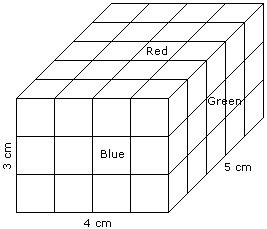# Verbal Reasoning - Cube and Cuboid - Discussion

The following questions are based on the information given below:

1. A cuboid shaped wooden block has 4 cm length, 3 cm breadth and 5 cm height.
2. Two sides measuring 5 cm x 4 cm are coloured in red.
3. Two faces measuring 4 cm x 3 cm are coloured in blue.
4. Two faces measuring 5 cm x 3 cm are coloured in green.
5. Now the block is divided into small cubes of side 1 cm each.

1.

How many small cubes will have will have three faces coloured ?

 [A]. 14 [B]. 8 [C]. 10 [D]. 12

Explanation:Such cubes are related to the corners of the cuboid and in the cuboid there are 8 corners.

Hence, the required number of small cubes is 8.

 Raj said: (Nov 27, 2011) In this cuboid why hight specifed 3 cm instead of 5cm?

 Karan said: (Nov 5, 2014) How can breadth be 5 cm?